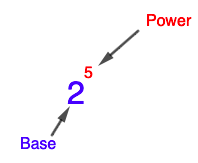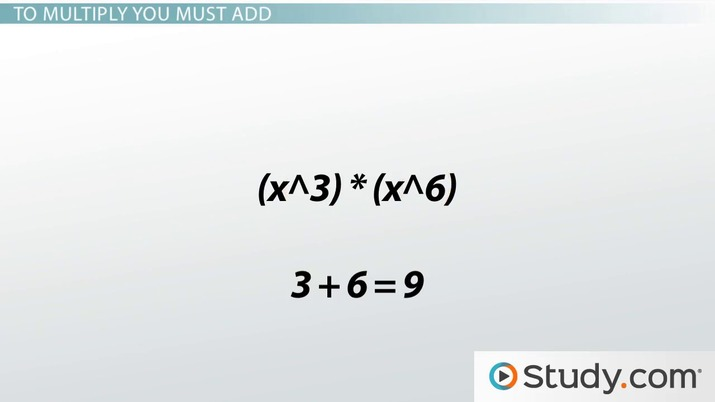Multiplying Expressions with Exponents

Stephen Sacchetti, Jennifer Beddoe
• Author
Stephen Sacchetti

Stephen graduated from Haverford College with a B.S. in Mathematics in 2011. For the past ten years, he has been teaching high school math and coaching teachers on best practices. In 2015, Stephen earned an M.S. Ed from the University of Pennsylvania where he currently works as an adjunct professor.

• Instructor
Jennifer Beddoe

Jennifer has an MS in Chemistry and a BS in Biological Sciences.

Learn about multiplying exponents with different bases, as well as multiplying exponents with the same base. Explore multiplying exponents rules, how to multiply exponents with different bases, and what happens when you multiply exponents. Updated: 11/08/2021

Show

How Do You Multiply Exponents?

This lesson will focus on operations on numbers that have exponents. In particular, the main goal of this lesson will be to answer the question of when and how do you multiply exponents. After establishing the when and how there will be an exploration of multiplying exponents rules that outline how to combine exponents in a variety of situations.

What are Exponents?

The four basic operations of arithmetic are addition, subtraction, multiplication, and division. Recall that multiplication is simply a shorter way to write repeated addition. For example, {eq}3 + 3 + 3 + 3 + 3 + 3 = 6 \times 3 {/eq}. Similarly, an exponent is an operation that is a shorter way to write repeated multiplication. For example, {eq}3 \times 3 \times 3 \times 3 \times 3 \times 3 = 3^6 {/eq}. In this case, 6 is called the exponent and 3 is called the base. At times, the word power is also used to describe an exponent. For instance, the expression in Fig. 1 might be called "two to the power of five."More generally, any exponential expression can be written as {eq}b^a {/eq} where a is the exponent and b is the base.An error occurred trying to load this video.

Try refreshing the page, or contact customer support.

Coming up next: Dividing Exponential Expressions

You're on a roll. Keep up the good work!

Replay
Your next lesson will play in 10 seconds
• 0:03 What Is an Exponent?
• 0:43 To Multiply You Must Add
• 2:25 What If the Terms Have…
• 3:33 Lesson Summary
Save Save

Want to watch this again later?

Timeline
Autoplay
Autoplay
Speed Speed

Multiplying Exponents with the Same Base: Do You Add Exponents When Multiplying?

What do you do when multiplying exponents? Since exponents mean repeated multiplication, a natural question of what happens when you multiply exponents might arise. In this section, the idea of multiplying two bases raised to exponents will be explored. For example, consider the expression {eq}2^5 \times 2^3 {/eq}. It may be tempting here to assume that the 2's multiply together or that the 5 and 3 multiply together, but in fact, neither of these is correct. Instead, lean on the definition of an exponent to rewrite this expression before combining any terms:

{eq}2^5 \times 2^3 = (2 \times 2 \times 2 \times 2 \times 2) \times (2 \times 2 \times 2) {/eq}

Noticing that there are eight total 2's here, this expression can be rewritten as {eq}2^8 {/eq}. This idea can be generalized to show that you add exponents when multiplying like bases: {eq}b^x \times b^y = b^{x + y} {/eq}

Multiplying Exponents With Different Bases

But what happens when multiplying exponents with different bases? This cannot be done by adding the exponents as in the previous section because it is unclear what to do with the base. This section will show how to multiply exponents with different bases using two different methods of simplification.

Exponential Multiplication: Simplification Method

The first method is the simplification method. Essentially, this method is used to write out the exponential expression as a long string of multiplication and then combine all of the terms. Consider the example: {eq}3^4 \times 4^3 {/eq}. Writing this out gives: {eq}3 \times 3 \times 3 \times 3 \times 4 \times 4 \times 4 {/eq} and none of the 3's can be combined with the 4's other than by multiplying out this product. Doing so gives an answer of 5184.

Exponential Multiplication: Grouping Method

The second method of multiplying exponential expressions with different bases is grouping. The grouping method essentially says to rearrange the terms so that the multiplication can be done a bit more easily. Recall the example above of {eq}3^4 \times 4^3 {/eq} Notice that there is one more 3 than there are 4's. So we can combine the same number of 3's and 4's and have one 3 left over. For instance:

{eq}3 \times 3 \times 3 \times 3 \times 4 \times 4 \times 4 = 3 \times 4 \times 3 \times 4 \times 3 \times 4 \times 3 = 12 \times 12 \times 12 \times 3 = 12^3 \times 3 {/eq}

So it is possible to rewrite the expression using exponents: {eq}3^4 \times 4^3 = 3^3 \times 4^3 \times 3 = 12^3 \times 3 {/eq}.

More generally, it can be said that {eq}a^x \times b^x = (ab)^x {/eq} or, in words, multiplying different bases raised to the same power means that the bases can be multiplied together first and then raised to that power.

Notice, though, that this grouping method requires having the same exponent on each base. For instance, to group {eq}x^5 \times y^7 {/eq}, first rewrite it as {eq}x^5 \times y^5 \times y^2 {/eq} and then group the terms with the same exponents: {eq}(xy)^5 \times y^2 {/eq}

Multiplying Exponents Rules: All Exponent Rules

There are seven rules to remember for multiplying bases with exponents. Each multiplying exponent rule will be shown below with an example to help illustrate it. Some of these rules do not involve multiplication but will be useful in solving more complicated problems involving multiplying exponents.

Rule 1: Product of Powers

If multiplying exponential expressions with the same base, then add the exponents together: {eq}2^4 \times 2^7 = 2^{11} {/eq}

To unlock this lesson you must be a Study.com Member.

Register to view this lesson

Are you a student or a teacher?